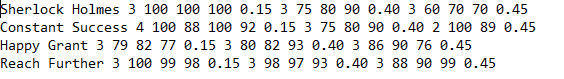# Sherlock Holmes 3 100 100 100 0.15 3 75 80 90 0.40 3 60 70 70 0.45Constant Success 4 100 88 100 92 0.15 3 75 80 90 0.40 2 100 89 0.45Happy Grant 3 79 82 77 0.15 3 80 82 93 0.40 3 86 90 76 0.45Reach Further 3 100 99 98 0.15 3 98 97 93 0.40 3 88 90 99 0.45

Question

c++ program to read file and output grades - file name is studentGrades.txt and the content is included in the image provided

code written so far (the middle portion is missing and will be included with the code from the following question):
_____________________________________________________________________

#include <iostream>
#include <fstream>
#include <iomanip>

using namespace std;

int main()
{
ifstream inFile; // This is a declaration of the inFile that holds all the grades
string fileName; // This is the filename that the user will enter
string name; // This is the name of the student
int gradeCount; // This is to keep count of the number of grades entered
int totalHWpoints; // Total homework points earned
int totalProgpoints; // Total program points earned
int totalExampoints; // Total exam points earned
int maxHWpoints; // The maximum points available for homework grades
int maxProgpoints; // The maximum points available for program grades
int maxExampoints; // The maximum points available for exam grades
float average; // The average of all the grades

cout << "Enter the input file name to read student grades: ";
cin >> fileName;

// Open the file with the grades

inFile.open(fileName.c_str ());

// Check to make sure the file opened correctly

if (!inFile)

{
cout << "File did not open correctly." << endl;
return 1;
}
while(HWscores >> score1 >> score2 >> score3);

// Calculate the average.
average = (score1 + score2 + score3) / 300;
cout << << average << endl; // Display the average.

if (average<90 && average >=80 ) finalGrade = 'B';
if (average<80 && average >=70) finalGrade = 'C';
if (average<70 && average >=60) finalGrade = 'D';

return 0;
}

______________________________________________________________

Using a looping construct, read until end of file. Do not hard code the number of rows in the loop.  The file must be read using an outer loop and three inner/nested loops to read all the data properly.  The first number will aid in determining the NUMBER of grades to read.  Do NOT assume there will be only 3 values in each category.  Code for a variable number of values in each category.

The maximum points for each assignment, homework and exam are 100. The maximum points for each may be calculated by multiplying the number of assignments in the group by 100.  Calculate final grade using the following formula:

((total HOMEWORK Points earned/maximum HOMEWORK points) * percent of total grade) + (total PROGRAM Points earned/maximum PROGRAM points) * percent of total grade) + (total EXAM Points earned/maximum EXAM points) * percent of total grade)) * 100help_outlineImage TranscriptioncloseSherlock Holmes 3 100 100 100 0.15 3 75 80 90 0.40 3 60 70 70 0.45 Constant Success 4 100 88 100 92 0.15 3 75 80 90 0.40 2 100 89 0.45 Happy Grant 3 79 82 77 0.15 3 80 82 93 0.40 3 86 90 76 0.45 Reach Further 3 100 99 98 0.15 3 98 97 93 0.40 3 88 90 99 0.45 fullscreen
check_circleExpert Solution
Step 1

Program Instructions:

• Comment the unused code and add necessary variables.
• Use a while and three inner while loops to get the data from file.
• For each entry show the final grade using the given formula.
Step 2

Program:
#include <iostream>

#include <fstream>

#include <iomanip>

using namespace std;

int main()

{

ifstream inFile; // This is a declaration of the inFile that holds all the grades

string fileName; // This is the filename that the user will enter

string name; // This is the name of the student

string surname;

int gradeCount; // This is to keep count of the number of grades entered

int totalHWpoints; // Total homework points earned

int totalProgpoints; // Total program points earned

int totalExampoints; // Total exam points earned

int maxHWpoints; // The maximum points available for homework grades

int maxProgpoints; // The maximum points available for program grades

int maxExampoints; // The maximum points available for exam grades

float average; // The average of all the grades

cout << "Enter the input file name to read student grades: ";

cin >> fileName;

// Open the file with the grades

inFile.open(fileName.c_str ());

// Check to make sure the file opened correctly

if (!inFile)

{

cout << "File did not open correctly." << endl;

return 1;

}

//while(HWscores >> score1 >> score2 >> score3){

//}

while(!inFile.eof()){

totalHWpoints=0;

totalProgpoints=0;

totalExampoints=0;

//   cout<<"hi";

//   getline(inFile,name);

inFile>>name;

inFile>>surname;

inF...

### Want to see the full answer?

See Solution

#### Want to see this answer and more?

Solutions are written by subject experts who are available 24/7. Questions are typically answered within 1 hour*

See Solution
*Response times may vary by subject and question
Tagged in

### Computer Science